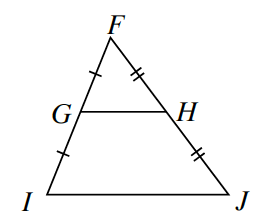### Home > CCG > Chapter 7 > Lesson 7.3.2 > Problem7-134

7-134.Examine the diagram at right.

1. Are the triangles in this diagram similar? If they are, use a flowchart with justifications or a two-column proof to prove similarity. If they are not similar, explain why not. Explain.

Do the marks help prove they are similar? If so, how? What makes these triangles similar? Are there any angles that are congruent?

Yes, by $\text{SAS}\sim$ because Segment $FI$ is proportional to segment $FG$, $∠F≅∠F$, and segment $FJ$ is proportional to segment $FH$.

2. Name all the pairs of congruent angles in this diagram you can.

Look at the triangles, what angles are corresponding to each other?

3. Are $\overline{GH}$ and $\overline{IJ}$ parallel? Explain how you know.

How can knowing sets of congruent angles, help you prove the sides are parallel?

Yes they are parallel, because corresponding angles are congruent.

4. If $GH=4x-3$ and $IJ=3x+14$, find $x$. Then find the length of $\overline{GH}$.

How are the lengths of $\overline{GH}$ and $\overline{IJ}$ related? How will this help you solve for $x$?